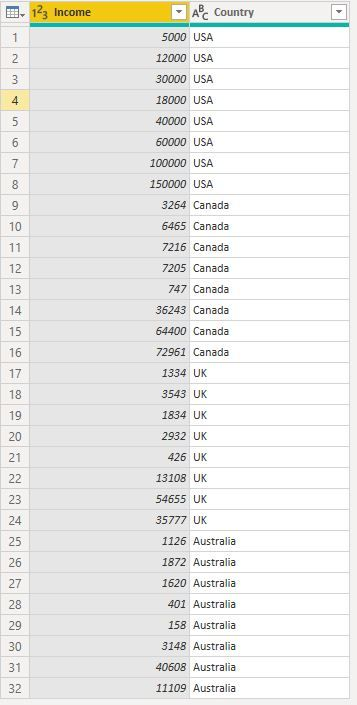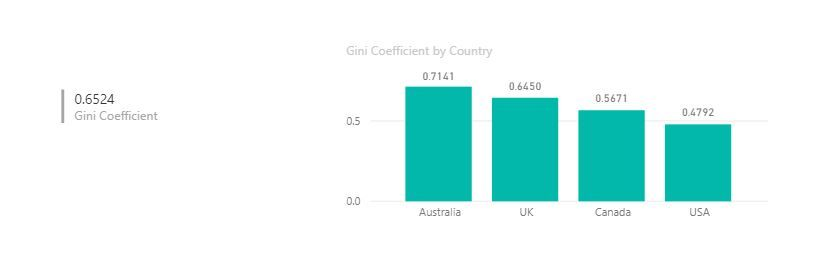cancel
Showing results for
Did you mean:

Fabric is Generally Available. Browse Fabric Presentations. Work towards your Fabric certification with the Cloud Skills Challenge.Frequent Visitor

## How to calculate GINI coefficient as a measure

Hi,

I'm wondering if it's possible to calculate the GINI coefficient as a measure.  I have data on panel studies which contains for each study ID the number of answers in that study. So basically one column for study IDs and another for the number of answers. These studies belong in different categories, although one study can belong in many categories at once.. I'd like to use the GINI coefficient to portray how evenly the answers are divided between studies, and then show this on a multi-row card for each category.

1 ACCEPTED SOLUTIONNew Member

Hi @palkamj,

This is definitely possible.

If we have a table ("IncomeTable") which contains a column with the data by individual:And if we add this measure to the data model:

(Note: adjust row 2 so that SELECTCOLUMNS points to the column that contains your data)

``````Gini Coefficient2 =
VAR DataColumn = SELECTCOLUMNS(IncomeTable,"Data",IncomeTable[Income])
VAR Areas_Under_Lorenz_Curve =
DataColumn,
"Area",
VAR IteratingIncome = [Data]
VAR LessOrEqual_Table =
FILTER ( DataColumn, [Data] <= IteratingIncome )
VAR LessOrEqualCummulative =
SUMX ( LessOrEqual_Table, [Data] )
VAR Less_Table =
FILTER ( DataColumn, [Data] < IteratingIncome )
VAR Less_Cummulative =
SUMX ( Less_Table, [Data] )
VAR TotalIncome =
SUMX ( DataColumn, [Data])
VAR NoOfDataPoints =
COUNTROWS ( DataColumn )
RETURN
DIVIDE (
DIVIDE ( LessOrEqualCummulative + Less_Cummulative, 2 ),
TotalIncome * NoOfDataPoints
)
)
RETURN
( 0.5 - SUMX ( Areas_Under_Lorenz_Curve, [Area] ) ) / 0.5``````

Then your report will calculate gini coefficients at any filter level:@v-shex-msft if you agree with please mark it as the solution to this question

3 REPLIES 3New Member

Hi @palkamj,

This is definitely possible.

If we have a table ("IncomeTable") which contains a column with the data by individual:And if we add this measure to the data model:

(Note: adjust row 2 so that SELECTCOLUMNS points to the column that contains your data)

``````Gini Coefficient2 =
VAR DataColumn = SELECTCOLUMNS(IncomeTable,"Data",IncomeTable[Income])
VAR Areas_Under_Lorenz_Curve =
DataColumn,
"Area",
VAR IteratingIncome = [Data]
VAR LessOrEqual_Table =
FILTER ( DataColumn, [Data] <= IteratingIncome )
VAR LessOrEqualCummulative =
SUMX ( LessOrEqual_Table, [Data] )
VAR Less_Table =
FILTER ( DataColumn, [Data] < IteratingIncome )
VAR Less_Cummulative =
SUMX ( Less_Table, [Data] )
VAR TotalIncome =
SUMX ( DataColumn, [Data])
VAR NoOfDataPoints =
COUNTROWS ( DataColumn )
RETURN
DIVIDE (
DIVIDE ( LessOrEqualCummulative + Less_Cummulative, 2 ),
TotalIncome * NoOfDataPoints
)
)
RETURN
( 0.5 - SUMX ( Areas_Under_Lorenz_Curve, [Area] ) ) / 0.5``````

Then your report will calculate gini coefficients at any filter level:@v-shex-msft if you agree with please mark it as the solution to this questionHelper III

Hello @Mikelg,
Thanks a lot for sharing,

when applying your formula to a simple test table

 Group Income A 500 B 0 C 0

The results obtain is 0.67 while it should be 1 as all income is detain by one group...
What surprise me is that nowhere in the formula we precise the grouping category
Let me know if i do something wrong or if any fixes need to be done to the formulaCommunity Support

HI @palkamj,

I don't think it is possible to achieve this requirement, may be your can take a look at below link which told about use excel to achieve gini coefficient.(include coding formula)

Fast Gini – calculating Gini coefficients in Excel efficiently

Regards,
Xiaoxin Sheng

Community Support Team _ Xiaoxin
If this post helps, please consider accept as solution to help other members find it more quickly.Announcements#### Power BI Monthly Update - November 2023

Check out the November 2023 Power BI update to learn about new features.#### Fabric Community News unified experience

Read the latest Fabric Community announcements, including updates on Power BI, Synapse, Data Factory and Data Activator.#### Exclusive opportunity for Women!

Join us for a free, hands-on Microsoft workshop led by women trainers for women where you will learn how to build a Dashboard in a Day!#### The largest Power BI and Fabric virtual conference

130+ sessions, 130+ speakers, Product managers, MVPs, and experts. All about Power BI and Fabric. Attend online or watch the recordings.Top Solution Authors
Top Kudoed Authors
Users online (7,429)